# Viewing Student Statistics

Student Records enables you to view summary information at various points during a student's academic career, with numerous ways to access the information. You can view summaries of both enrollment and term statistics.

This section discusses how to:

• View student enrollment summaries.

• View term statistics.

• Calculate term statistic values.

• View cumulative statistics for multiple terms.

• Calculate cumulative statistic values.

• View student terms.

Page Name

Definition Name

Usage

Enrollment Summary

STDNT_ENRL_INQ

select Records and Enrollment, then select Enrollment Summaries, then select Enrollment Summary, then select Enrollment Summary

View a summary of enrollment information. The student must first enroll in classes.

Enrollment Summary - Term Statistics

TERM_STATISTICS2

select Records and Enrollment, then select Enrollment Summaries, then select Enrollment Summary, then select Term Statistics

View term statistics for a specific term. The student must first enroll in classes, have posted transfer credits, or both.

Term History - Term Statistics

TERM_STATISTICS

select Records and Enrollment, then select Student Term Information, then select Term History, then select Term Statistics

View term statistics for all terms within an academic career. The student must first enroll in classes, have posted transfer credits, or both.

Cumulative Statistics

CUM_STATISTICS

select Records and Enrollment, then select Student Term Information, then select Term History, then select Cumulative Statistics

View cumulative statistics for all terms within an academic career. The student must first enroll in classes, have posted transfer credits, or both.

Student Term Search

STDNT_TERM_SRCH

select Records and Enrollment, then select Career and Program Information, then select Student Term Search, then select Student Term Search

View all terms in which a student has been active.

Access the Enrollment Summary page (select Records and Enrollment, then select Enrollment Summaries, then select Enrollment Summary, then select Enrollment Summary).

Image: Enrollment Summary page

This example illustrates the fields and controls on the Enrollment Summary page. You can find definitions for the fields and controls later on this page.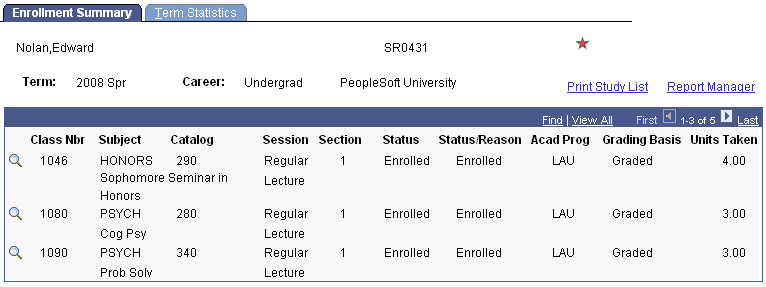View all classes in which a student is successfully enrolled for the term.

Field or Control

Definition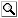Click the Course Detail button to access the Class Detail page, where you can view details about the class listed on the Enrollment Summary page.

Print Study List

Click to print the student's enrollment summary.

Access the Term History - Term Statistics page (select Records and Enrollment, then select Student Term Information, then select Term History, then select Term Statistics).

Image: Term History - Term Statistics page (1 of 2)

This example illustrates the fields and controls on the Term History - Term Statistics page (1 of 2). You can find definitions for the fields and controls later on this page.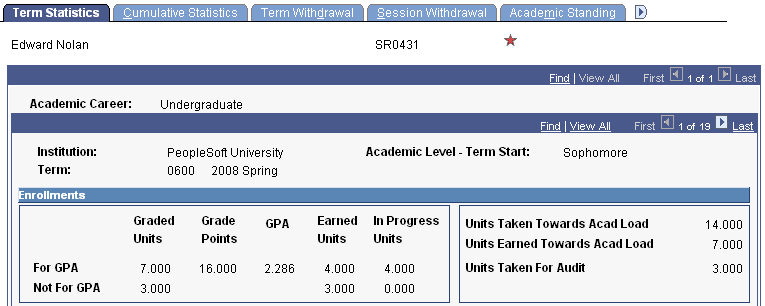Image: Term History - Term Statistics page (2 of 2)

This example illustrates the fields and controls on the Term History - Term Statistics page (2 of 2). You can find definitions for the fields and controls later on this page.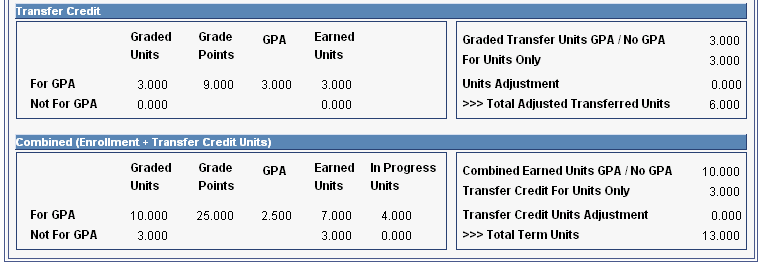View enrollment and transfer credit statistics for all terms in which the student has been or is enrolled. You can view this information for an individual term in the Enrollment Summary component.

### Summary of Student Enrollment for the Term

The following information refers to the example screen shot:

• 4 Units with Grade A (for GPA) – 16 grade points

• 3 Units with Grade F (for GPA) – 0 grade points

• 3 Units with Grade Pass (Not For GPA)

• 4 Units with no grade (in progress) (class will be For GPA after grading)

• 3 Units with an Audit grade basis

### Enrollments

Field or Control

Definition

Displays the total number of units that are taken for a grade (A, B, C, D, F) and accumulate in the GPA.

Displays the total number of units that are taken for a grade (Pass, Fail, Satisfactory, Unsatisfactory) and do not accumulate in the GPA.

Note: The Graded Units - For GPA and Graded Units - Not for GPA fields do not include classes that are in a withdrawn status and classes that are taken with the audit grade basis.

Displays a number that is calculated by taking the grade points received for each grade (on a 4-point grading scale, an A equals 4 points), and multiplying that number by the number of units taken for each class for which a grade accumulates in the GPA.

GPA - For GPA (grade point average - for grade point average)

Displays the grade point average which is calculated by dividing the grade points for GPA by the graded units for GPA.

Use the GPA Rounding/Truncating Option field on the Student Records Installation page (Set Up SACR, Install, Student Records Installation) to indicate to how many decimal places you want to round or truncate the grade point average.

Note: No values appear in the Not For GPA row for the Grade Points and GPA fields.

Earned Units - For GPA (earned units - for grade point average)

Displays the total number of units that are passed with an earned credit grade (A, B, C, D) and accumulate in the GPA.

Earned Units - Not For GPA (earned units - not for grade point average)

Displays the total number of units that are passed with an earned credit grade (Pass, Satisfactory) and do not accumulate in the GPA.

In Progress Units - For GPA (in progress units - for grade point average)

Displays the total number of units that are not yet completed and for which the future assigned grade will accumulate in the GPA.

In Progress Units - Not For GPA (in progress units - not for grade point average)

Displays the total number of units that are not yet completed and for which the future assigned grade will not accumulate in the GPA.

Displays the total number of units taken for progress. This total is used in Student Records to determine academic load.

Displays the total number of units passed for progress. This total is used in Student Records to determine academic load and academic level.

Units Taken For Audit

Displays the total number of audit units taken for the term.

### Summary of Student Transfer Credit for Term

The following information refers to the example screen shot:

• 3 Units with Grade B (for GPA) for a model where the Include in GPA check box is selected on the Transfer Course Entry page.

• 3 Units with Grade T for a model where the Include in GPA check box is cleared on the Transfer Course Entry page.

### Transfer Credit

The statistics in this group box include both internal and external transfer credits.

Field or Control

Definition

Displays the total number of units that are transferred for a grade (A, B, C, D, F) and accumulate in the GPA for models where the Include in GPA check box is selected on the Transfer Course Entry page.

Displays the total number of units that are transferred for a grade (T) and do not accumulate in the GPA for models where the Include in GPA check box is selected on the Transfer Course Entry page.

Displays a number that is calculated by taking the grade points received for each grade (on a 4-point grading scale, an A equals 4 points), and multiplying that number by the number of units taken for each transferred class for which a grade accumulates in the GPA.

GPA - For GPA (grade point average - for grade point average)

Displays the grade point average which is calculated by dividing the grade points for GPA by the graded units for GPA.

Use the GPA Rounding/Truncating Option field on the Student Records Installation page (Set Up SACR, Install, Student Records Installation) to indicate to how many decimal places you want to round or truncate the grade point average.

Note: No values appear in the Not For GPA row for the Grade Points and GPA fields.

Earned Units - For GPA (earned units - for grade point average)

Displays the total number of transferred units that are passed with an earned credit grade (A, B, C, D) and accumulate in the GPA for models where the Include in GPA check box is selected on the Transfer Course Entry page.

Earned Units - Not For GPA (earned units - not for grade point average)

Displays the total number of transferred units that are passed with an earned credit grade (T) and do not accumulate in the GPA for models where the Include in GPA check box is selected on the Transfer Course Entry page.

Displays the sum of transferred units for which received grades both accumulate and do not accumulate in the GPA for models where the Include in GPA check box is selected on the Transfer Course Entry page.

For Units Only

Displays the total number of transferred units that are passed with an earned credit grade and do not accumulate in the GPA for models where the Include in GPA check box is cleared on the Transfer Course Entry page.

Displays the total number of units that were manually removed from the student's overall transfer credit units. This field is updated on the Terms in Residence page in the Term Activation component.

Note: This number displays as a positive value, but is stored as a negative value.

Displays the sum of graded transfer units (for GPA and not for GPA), plus the value in the Units Only field, minus the value in the Units Adjustment field.

### Combined (Enrollment + Transfer Credit Units)

Field or Control

Definition

Displays the sum of all enrollment and transfer credit units that are taken and transferred for a grade (A, B, C, D, F) and accumulate in the GPA.

Displays the sum of all enrollment and transfer credit units that are taken and transferred for a grade (Pass, Fail, Satisfactory, Unsatisfactory, T) and do not accumulate in the GPA.

Displays the sum of all grade points for enrollment and transfer credit units that accumulate in the GPA.

GPA - For GPA (grade point average - for grade point average)

Displays the grade point average which is calculated by dividing the grade points for GPA by the graded units for GPA.

Use the GPA Rounding/Truncating Option field on the Student Records Installation page (Set Up SACR, Install, Student Records Installation) to indicate to how many decimal places you want to round or to truncate the grade point average.

Note: No values appear in the Not For GPA row for the Grade Points and GPA fields.

Earned Units - For GPA (earned units - for grade point average)

Displays the sum of all enrollment and transfer credit units that are passed with an earned credit grade ( A, B, C, D) and accumulate in the GPA.

Earned Units - Not For GPA (earned units - not for grade point average)

Displays the sum of all enrollment and transfer credit units that are passed with an earned credit grade (Pass, Satisfactory, T) and do not accumulate in the GPA.

In Progress Units - For GPA (in progress units - not for grade point average)

Displays the total number of units that are not yet completed and for which the future assigned grade will accumulate in the GPA.

In Progress Units - Not For GPA (in progress units - not for grade point average)

Displays the total number of units that are not yet completed and for which the future assigned grade will not accumulate in the GPA.

Combined Earned Unit GPA/ No GPA (combined earned unit for grade point average / not for grade point average)

Displays the sum of all enrollment and transfer credit units that are passed with an earned credit grade, including both those that accumulate and do not accumulate in the GPA.

Transfer Credit For Units Only

Displays the sum of transferred units that are passed with an earned credit grade and do not accumulate in the GPA for models where the Include in GPA check box is cleared on the Transfer Course Entry page.

Displays the number of units that were manually removed from the student's overall transfer credit units.

Total Term Units

Displays the sum of all enrollment and transfer credit units that are passed with an earned credit grade, including both those that accumulate and do not accumulate in the GPA, plus transfer credit units that are passed with an earned credit grade that do not accumulate in the GPA for models where the Include in GPA check box is cleared, minus the number of units that were manually removed from the student's overall transfer credit units. Units taken for audit are excluded from this total.

Use the following table to understand how term statistic values are calculated.

Term Statistics Page Field

Field from STDNT_CAR_TERM

Calculation

ENROLLMENT

UNT_TAKEN_GPA

Sum of UNT_TAKEN from STDNT_ENRL where

UNITS_ATTEMPTED = Y,

INCLUDE_IN_GPA = Y,

EARN_CREDIT = n/a

Graded Units - Not For GPA

UNT_TAKEN_NOGPA

Sum of UNT_TAKEN from STDNT_ENRL where

UNITS_ATTEMPTED = Y,

INCLUDE_IN_GPA = N,

EARN_CREDIT = n/a

Sum of GRADE_POINTS from STDNT_ENRL where

UNITS_ATTEMPTED = Y,

INCLUDE_IN_GPA = Y,

EARN_CREDIT = n/a

GPA - For GPA

CUR_GPA

Earned Units - For GPA

UNT_PASSD_GPA

Sum of UNT_EARNED from STDNT_ENRL where

UNITS_ATTEMPTED = Y,

INCLUDE_IN_GPA = Y,

EARN_CREDIT = Y

Earned Units - Not For GPA

UNT_PASSD_NOGPA

Sum of UNT_EARNED from STDNT_ENRL where

UNITS_ATTEMPTED = Y,

INCLUDE_IN_GPA = N,

EARN_CREDIT = Y

In-Progress Units - For GPA

UNT_INPROG_GPA

Sum of UNT_TAKEN from STDNT_ENRL where

UNITS_ATTEMPTED = I,

INCLUDE_IN_GPA = Y,

EARN_CREDIT = n/a

In-Progress Units - Not For GPA

UNT_INPROG_NOGPA

Sum of UNT_TAKEN from STDNT_ENRL where

UNITS_ATTEMPTED = I,

INCLUDE_IN_GPA = N,

EARN_CREDIT = n/a

UNT_TAKEN_GPA + UNT_TAKEN_NOGPA + UNT_INPROG_GPA + UNT_INPROG_NOGPA

Sum of UNT_PRGRSS from STDNT_ENRL where

UNITS_ATTEMPTED = I or Y,

INCLUDE_IN_GPA = n/a,

EARN_CREDIT = n/a

UNT_PASSD_GPA+ UNT_PASSD_NOGPA

Sum of UNT_PRGRSS from STDNT_ENRL where

UNITS_ATTEMPTED = Y,

INCLUDE_IN_GPA = Y,

EARN_CREDIT = Y

Units Taken For Audit

UNT_AUDIT

Sum of UNT_PRGRSS from STDNT_ENRL where

UNITS_ATTEMPTED = n/a,

INCLUDE_IN_GPA = n/a,

EARN_CREDIT = n/a

TRANSFER CREDIT

TRF_TAKEN_GPA

Course Credits:

1. Internal Transfer (TRNS_CRSE_SCH.TRNSFR_SRC_TYPE = I)

Sum of UNIT_TRNSFR from TRNS_CRSE_DTL where

UNITS_ATTEMPTED = Y,

INCLUDE_IN_GPA = Y,

EARN_CREDIT = n/a

2. External Transfer (TRNS_CRSE_SCH.TRNSFR_SRC_TYPE =E)

Sum of UNIT_TRNSFR from TRNS_CRSE_DTL where

ORG_INST_TBL.TRNSFR_CR_INCL_GPA = Y,

UNITS_ATTEMPTED = Y,

INCLUDE_IN_GPA = Y,

EARN_CREDIT = n/a

3. Manual Transfer (TRNS_CRSE_SCH.TRNSFR_SRC_TYPE =M)

Sum of UNIT_TRNSFR from TRNS_CRSE_DTL where

TRNS_CRSE_SCH.INCLUDE_IN_GPA = Y,

UNITS_ATTEMPTED = Y,

INCLUDE_IN_GPA = Y,

EARN_CREDIT = n/a

Test Credits:

Sum of UNIT_TRNSFR from TRNS_TEST_DTL where

TRNS_TEST_MODEL.INCLUDE_IN_GPA = Y,

UNITS_ATTEMPTED = Y,

INCLUDE_IN_GPA = Y,

EARN_CREDIT = n/a

Other Credits:

Sum of UNIT_TRNSFR from TRNS_OTHR_DTL where

TRNS_OTHR_MODEL.INCLUDE_IN_GPA = Y,

UNITS_ATTEMPTED = Y,

INCLUDE_IN_GPA = Y,

EARN_CREDIT = n/a

Graded Units - Not For GPA

TRF_TAKEN_NOGPA

Course Credits:

1. Internal Transfer (TRNS_CRSE_SCH.TRNSFR_SRC_TYPE = I)

Sum of UNIT_TRNSFR from TRNS_CRSE_DTL where

UNITS_ATTEMPTED = Y,

INCLUDE_IN_GPA = N,

EARN_CREDIT = n/a

2. External Transfer (TRNS_CRSE_SCH.TRNSFR_SRC_TYPE =E)

Sum of UNIT_TRNSFR from TRNS_CRSE_DTL where

ORG_INST_TBL.TRNSFR_CR_INCL_GPA = Y,

UNITS_ATTEMPTED = Y,

INCLUDE_IN_GPA = N,

EARN_CREDIT = n/a

3. Manual Transfer (TRNS_CRSE_SCH.TRNSFR_SRC_TYPE =M)

Sum of UNIT_TRNSFR from TRNS_CRSE_DTL where TRNS_CRSE_SCH.INCLUDE_IN_GPA = Y,

UNITS_ATTEMPTED = Y,

INCLUDE_IN_GPA = N,

EARN_CREDIT = n/a

Test Credits:

Sum of UNIT_TRNSFR from TRNS_TEST_DTL where

TRNS_TEST_MODEL.INCLUDE_IN_GPA = Y,

UNITS_ATTEMPTED = Y,

INCLUDE_IN_GPA = N,

EARN_CREDIT = n/a

Other Credits:

Sum of UNIT_TRNSFR from TRNS_OTHR_DTL where

TRNS_OTHR_MODEL.INCLUDE_IN_GPA = Y,

UNITS_ATTEMPTED = Y,

INCLUDE_IN_GPA = N,

EARN_CREDIT = n/a

Course Credits:

1. Internal Transfer (TRNS_CRSE_SCH.TRNSFR_SRC_TYPE = I)

Sum of (UNIT_TRNSFR * GRD_PTS_PER_UNIT) from TRNS_CRSE_DTL where

UNITS_ATTEMPTED = Y,

INCLUDE_IN_GPA = Y,

EARN_CREDIT = n/a

2. External Transfer (TRNS_CRSE_SCH.TRNSFR_SRC_TYPE =E)

Sum of (UNIT_TRNSFR * GRD_PTS_PER_UNIT) from TRNS_CRSE_DTL where

ORG_INST_TBL.TRNSFR_CR_INCL_GPA = Y,

UNITS_ATTEMPTED = Y,

INCLUDE_IN_GPA = Y,

EARN_CREDIT = n/a

3. Manual Transfer (TRNS_CRSE_SCH.TRNSFR_SRC_TYPE =M)

Sum of (UNIT_TRNSFR * GRD_PTS_PER_UNIT) from TRNS_CRSE_DTL where

TRNS_CRSE_SCH.INCLUDE_IN_GPA = Y,

UNITS_ATTEMPTED = Y,

INCLUDE_IN_GPA = Y,

EARN_CREDIT = n/a

Test Credits:

Sum of (UNIT_TRNSFR * GRD_PTS_PER_UNIT) from TRNS_TEST_DTL where

TRNS_TEST_MODEL.INCLUDE_IN_GPA = Y,

UNITS_ATTEMPTED = Y,

INCLUDE_IN_GPA = Y,

EARN_CREDIT = n/a

Other Credits:

Sum of (UNIT_TRNSFR * GRD_PTS_PER_UNIT) from TRNS_OTHR_DTL where

TRNS_OTHR_MODEL.INCLUDE_IN_GPA = Y,

UNITS_ATTEMPTED = Y,

INCLUDE_IN_GPA = Y,

EARN_CREDIT = n/a

GPA - For GPA

SSR_TRF_CUR_GPA

Earned Units - For GPA

TRF_PASSED_GPA

Course Credit

1. Internal Transfer (TRNS_CRSE_SCH.TRNSFR_SRC_TYPE = I)

Sum of UNIT_TRNSFR from TRNS_CRSE_DTL where

UNITS_ATTEMPTED = Y,

INCLUDE_IN_GPA = Y,

EARN_CREDIT = Y

2. External Transfer (TRNS_CRSE_SCH.TRNSFR_SRC_TYPE =E)

Sum of UNIT_TRNSFR from TRNS_CRSE_DTL where

ORG_INST_TBL.TRNSFR_CR_INCL_GPA = Y,

UNITS_ATTEMPTED = Y,

INCLUDE_IN_GPA = Y,

EARN_CREDIT = Y

3. Manual Transfer (TRNS_CRSE_SCH.TRNSFR_SRC_TYPE =M)

Sum of UNIT_TRNSFR from TRNS_CRSE_DTL where

TRNS_CRSE_SCH.INCLUDE_IN_GPA = Y,

UNITS_ATTEMPTED = Y,

INCLUDE_IN_GPA = Y,

EARN_CREDIT = Y

Test Credits:

Sum of UNIT_TRNSFR from TRNS_TEST_DTL where

TRNS_TEST_MODEL.INCLUDE_IN_GPA = Y,

UNITS_ATTEMPTED = Y,

INCLUDE_IN_GPA = Y,

EARN_CREDIT = Y

Other Credits:

Sum of UNIT_TRNSFR from TRNS_OTHR_DTL where

TRNS_OTHR_MODEL.INCLUDE_IN_GPA = Y,

UNITS_ATTEMPTED = Y,

INCLUDE_IN_GPA = Y,

EARN_CREDIT = Y

Earned Units - Not For GPA

TRF_PASSED_NOGPA

Course Credits:

1. Internal Transfer (TRNS_CRSE_SCH.TRNSFR_SRC_TYPE = I)

Sum of UNIT_TRNSFR from TRNS_CRSE_DTL where

UNITS_ATTEMPTED = Y,

INCLUDE_IN_GPA = N,

EARN_CREDIT = Y

2. External Transfer (TRNS_CRSE_SCH.TRNSFR_SRC_TYPE =E)

Sum of UNIT_TRNSFR from TRNS_CRSE_DTL where

ORG_INST_TBL.TRNSFR_CR_INCL_GPA = Y,

UNITS_ATTEMPTED = Y,

INCLUDE_IN_GPA = N,

EARN_CREDIT = Y

3. Manual Transfer (TRNS_CRSE_SCH.TRNSFR_SRC_TYPE =M)

Sum of UNIT_TRNSFR from TRNS_CRSE_DTL where

TRNS_CRSE_SCH.INCLUDE_IN_GPA = Y,

UNITS_ATTEMPTED = Y,

INCLUDE_IN_GPA = N,

EARN_CREDIT = Y

Test Credits:

Sum of UNIT_TRNSFR from TRNS_TEST_DTL where

TRNS_TEST_MODEL.INCLUDE_IN_GPA = Y,

UNITS_ATTEMPTED = Y,

INCLUDE_IN_GPA = N,

EARN_CREDIT = Y

Other Credits:

Sum of UNIT_TRNSFR from TRNS_OTHR_DTL where

TRNS_OTHR_MODEL.INCLUDE_IN_GPA = Y,

UNITS_ATTEMPTED = Y,

INCLUDE_IN_GPA = N,

EARN_CREDIT = Y

Graded Transferred Units GPA / No GPA

DERIVED

TRF_TAKEN_GPA+ TRF_TAKEN_NOGPA

For Units Only

DERIVED

UNT_TRNSFR + UNT_TEST_CREDIT + UNT_OTHER

As entered on Terms of Residence page

DERIVED

(TRF_TAKEN_GPA+ TRF_TAKEN_NOGPA) + (UNT_TRNSFR + UNT_TEST_CREDIT + UNT_OTHER) - TC_UNITS_ADJUST

COMBINED UNITS

DERIVED

UNT_TAKEN_GPA

+ TRF_TAKEN_GPA

Graded Units - Not For GPA

DERIVED

UNT_TAKEN_NOGPA+ TRF_TAKEN_NOGPA

DERIVED

GPA - For GPA

SSR_COMB_CUR_GPA

Earned Units - For GPA

DERIVED

UNT_PASSD_GPA + TRF_PASSED_GPA

Earned Units - Not for GPA

DERIVED

UNT_PASSD_NOGPA+ TRF_PASSED_NOGPA

In Progress Units - For GPA

UNT_INPROG_GPA

Sum of UNT_TAKEN from STDNT_ENRL where

UNITS_ATTEMPTED = I,

INCLUDE_IN_GPA = Y,

EARN_CREDIT = n/a

In Progress Units - Not For GPA

UNT_INPROG_NOGPA

Sum of UNT_TAKEN from STDNT_ENRL where

UNITS_ATTEMPTED = I,

INCLUDE_IN_GPA = N,

EARN_CREDIT = n/a

Combined Earned Unit GPA/ No GPA

DERIVED

(UNT_PASSD_GPA + TRF_PASSED_GPA) +

(UNT_PASSD_NOGPA + TRF_PASSED_NOGPA)

Transfer Credit For Units Only

DERIVED

UNT_TRNSFR + UNT_TEST_CREDIT + UNT_OTHER

As entered on Terms of Residence page

Total Term Units

UNT_TERM_TOT

((UNT_PASSD_GPA + TRF_PASSED_GPA) + (UNT_PASSD_NOGPA + TRF_PASSED_NOGPA)) + (UNT_TRNSFR + UNT_TEST_CREDIT + UNT_OTHER) - TC_UNITS_ADJUST

Access the Cumulative Statistics page (select Records and Enrollment, then select Student Term Information, then select Term History, then select Cumulative Statistics).

Image: Cumulative Statistics page (1 of 2)

This example illustrates the fields and controls on the Cumulative Statistics page (1 of 2). You can find definitions for the fields and controls later on this page.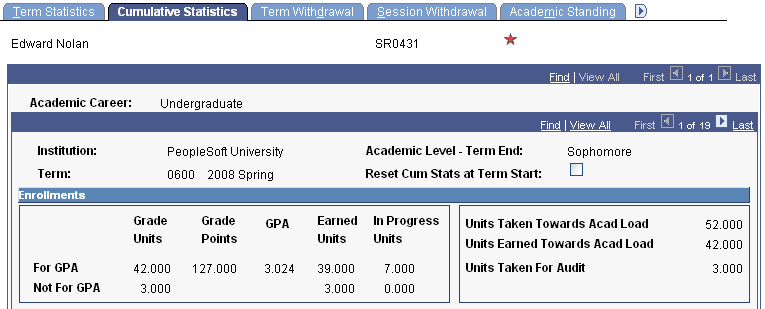Image: Cumulative Statistics page (2 of 2)

This example illustrates the fields and controls on the Cumulative Statistics page (2 of 2). You can find definitions for the fields and controls later on this page.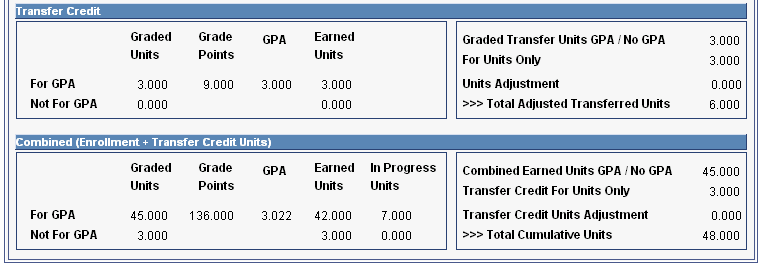### Enrollments

Field or Control

Definition

Units Taken for Audit

Displays the total cumulative audit units taken.

Note: All other field definitions are the same as the definitions for the fields in the Enrollments group box on the Term Statistics page.

Note: RESET_CUM_STATS triggers a reset in cumulative computation.

### Transfer Credit

All field definitions are the same as the definitions for the fields in the Transfer Credit group box on the Term Statistics page.

### Combined (Enrollment + Transfer Credit Units)

Field or Control

Definition

Total Cumulative Units

Displays the sum of all enrollment and transfer credit units that are passed with an earned credit grade, including both those accumulated and not accumulated in the GPA, plus transfer credit units that are passed with an earned credit grade and do not accumulate in the GPA for models where Include in GPA check box is cleared, minus the number of units that were manually removed from the student's overall transfer credit units. Units taken for audit are excluded from this total.

All other field definitions are the same as the definitions for the fields in the Combined (Enrollment + Transfer Credit Units) group box on the Term Statistics page.

Use the following table to understand how cumulative statistic values are calculated.

Cumulative Statistics Page Field

Field from STDNT_CAR_TERM

Calculation

ENROLLMENT

SSR_TOT_EN_TKN_GPA

Cumulative sum of UNT_TAKEN_GPA

Graded Units - Not For GPA

DERIVED

Cumulative sum of UNT_TAKEN_NOGPA

SSR_TOT_EN_GRDPTS

GPA - For GPA

SSR_CUM_EN_GPA

SSR_TOT_EN_GRDPTS / SSR_TOT_EN_TKNGPA

Earned Units - For GPA

DERIVED

Cumulative sum of UNT_PASSD_GPA

Earned Units - Not For GPA

DERIVED

Cumulative sum of UNT_PASSD_NOGPA

In-Progress Units - For GPA

TOT_INPROG_GPA

Cumulative sum of UNT_INPROG_GPA

In-Progress Units - Not For GPA

TOT_INPROG_NOGPA

Cumulative sum of UNT_INPROG_NOGPA

DERIVED

DERIVED

Units Taken For Audit

TOT_AUDIT

Cumulative sum of UNT_AUDIT

TRANSFER CREDIT

SSR_TOT_TR_TKNGPA

Cumulative sum of TRF_TAKEN_GPA

Graded Units - Not For GPA

DERIVED

Cumulative sum of TRF_TAKEN_NOGPA

SSR_TOT_TR_GRDPTS

GPA - For GPA

SSR_CUM_TR_GPA

SSR_TOT_TR_GRDPTS / SSR_TOT_TR_TKNGPA

Earned Units - For GPA

DERIVED

Cumulative sum of TRF_PASSED_GPA

Earned Units - Not For GPA

DERIVED

Cumulative sum of TRF_PASSED_NOGPA

Graded Transferred Units GPA / No GPA

DERIVED

SSR_TOT_TR_TKNGPA+ Cumulative sum of TRF_TAKEN_NOGPA

For Units Only

DERIVED

TOT_TRNSFR + TOT_TEST_CREDIT + TOT_OTHER

DERIVED

DERIVED

SSR_TOT_TR_TKNGPA+ Cumulative sum of TRF_TAKEN_NOGPA) + (TOT_TRNSFR + TOT_TEST_CREDIT + TOT_OTHER) – Cumulative sum of TC_UNITS_ADJUST

COMBINED UNITS

TOT_TAKEN_GPA

SSR_TOT_EN_TKN_GPA + SSR_TOT_TR_TKNGPA

Graded Units - Not For GPA

TOT_TAKEN_NOGPA

Cumulative sum of UNT_TAKEN_NOGPA + Cumulative sum of TRF_TAKEN_NOGPA

SSR_TOT_EN_GRDPTS + SSR_TOT_TR_GRDPTS

GPA - For GPA

CUM_GPA

(SSR_TOT_EN_GRDPTS + SSR_TOT_TR_GRDPTS) / (SSR_TOT_EN_TKN_GPA + SSR_TOT_TR_TKNGPA)

Earned Units - For GPA

TOT_PASSD_GPA

Cumulative sum of UNT_PASSD_GPA + Cumulative sum of TRF_PASSED_GPA

Earned Units - Not for GPA

TOT_PASSD_NOGPA

Cumulative sum of UNT_PASSD_NOGPA + Cumulative sum of TRF_PASSED_NOGPA

In Progress Units - For GPA

TOT_INPROG_GPA

Cumulative sum of UNT_INPROG_GPA

In Progress Units - Not For GPA

TOT_INPROG_NOGPA

Cumulative sum of UNT_INPROG_NOGPA

Combined Earned Unit GPA/ No GPA

DERIVED

(Cumulative sum of UNT_PASSD_GPA + Cumulative sum of TRF_PASSED_GPA) + (Cumulative sum of UNT_PASSD_NOGPA + Cumulative sum of TRF_PASSED_NOGPA)

Transfer Credit For Units Only

DERIVED

TOT_TRNSFR + TOT_TEST_CREDIT + TOT_OTHER

DERIVED

Total Cumulative Units

TOT_CUMULATIVE

Sum of TOT_PASSD_GPA + TOT_PASSD_NOGPA + TOT_TRNSFR +TOT_TEST_CREDIT + TOT_OTHER – (Cumulative Sum of TC_UNITS_ADJUST)

Access the Student Term Search page (select Records and Enrollment, then select Career and Program Information, then select Student Term Search, then select Student Term Search).

Field or Control

Definition

Every academic career for which the student has been active for a term.

Term

Every term in which the student has been active.